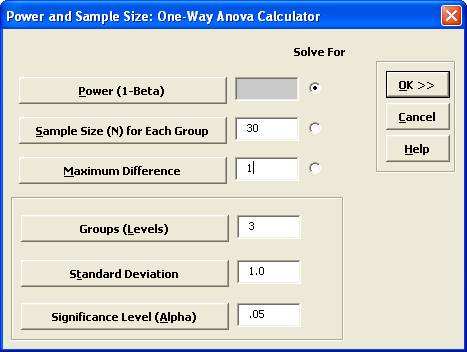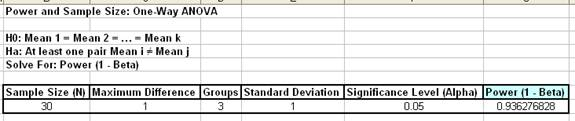Include Top

# How do I perform Power and Sample Size calculations for One-Way Anova?

## Power & Sample Size for One-Way ANOVA

To determine Power & Sample Size for a One-Way ANOVA, you can use the Power & Sample Size Calculator or Power & Sample Size with Worksheet.

1. Click SigmaXL > Statistical Tools > Power & Sample Size Calculators > One-Way ANOVA Calculator.
2. Select Solve For Power (1 – Beta). Enter Sample Size and Maximum Difference as shown:Note that we are calculating the power or likelihood of detection given that the maximum difference between group means = 1, with sample size for each group = 30, 3 groups, standard deviation = 1, significance level = .05, and Ha: Not Equal To (two sided test).

3. Click OK. The resulting report is displayed:

4.A power value of 0.94 is acceptable. Note that this value is less than the power value of 0.97 obtained with the two-sample t-Test.

5. Press F3 or click Recall SigmaXL Dialog to recall last dialog. Change the number of groups to 4. Note that the power value is 0.907. If the number of groups (levels) increases, you will have to increase the sample size in order to maintain statistical power.
6. To determine Power & Sample Size using a Worksheet, click SigmaXL > Statistical Tools > Power & Sample Size with Worksheet > One-Way ANOVA.
7. A graph showing the relationship between Power, Sample Size and Maximum Difference can then be created using SigmaXL > Statistical Tools > Power & Sample Size Chart. See here for an example using the 1 Sample t-Test.

# Web Demos

Our CTO and Co-Founder, John Noguera, regularly hosts free Web Demos featuring SigmaXL and DiscoverSim